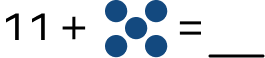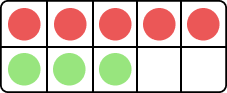# What is Count On in Maths? Definition, Meaning, Strategy, Example

Home » Math-Vocabluary » What is Count On in Maths? Definition, Meaning, Strategy, Example

## What is Count On?

Count on in maths is a mental math strategy used to add numbers. Using this technique, a student starts with the larger number and “counts on” with the other addends to get to the sum.

For example, if the number sentence is 4 + 3, the student will identify 4 as the larger number and count on three more—“4 … 5, 6, 7”. The answer or the sum is 7. Using the count on math fact, students have to be able to “hold” a number in their minds and then add on to it.

## How Can This Technique Be Taught?

Initially, when asked to find the total objects in two sets, young pupils may have to count both sets. This method is called “count all”. Usually, kids use the count all strategy to add when they can not visualize what number a group or set represents.

Students start with a pre-determined number in the count on strategy and count up. They can work their way up to counting from larger numbers. It is also vital for children to understand the terms “bigger number” and “smaller number” when counting on. In an example of count on math fact, if they are presented with the operation 4 + 7, they must identify 7 as the bigger number, begin counting up 4 from 7, and stop at 11.

A few ways to reinforce this strategy are as below.

## Dot Pattern

This technique, encourage students to identify the bigger number and then start counting on from there using the dots.

For example, 7 + 3

7 and counting on 3 dots as 8, 9, 10. So, the sum is 10.

## Number line

Step 1: Identify and locate the larger addend on the number line.

Step 2: Use “jumps” to count the smaller addend.

## Real-life Application of the Concept

Addition by counting on can be applied to various daily tasks such as setting the table, choosing items at the store, and participating in several games.

Counting is not effective for complex addition procedures. With time, the concept will become a part of students’ “addition toolkit.” However, it is an efficient starting approach for kids in their early elementary school years.

## Conclusion

The count-on technique is valuable and interesting for the students to learn addition.

## Solved Examples

Example 1: Find the sum by counting on.

Solution

Start counting 3 more from 7 as 8, 9, 10.

So, the sum is 10.

Example 2: Using the count on strategy, find the sum.

Solution:

There are 4 dots.

Start counting 4 more from 12 using the dots as 13, 14, 15, 16.

So, 12 + 4 = 16

Example 3: Find the missing number using the number line.

8 + ____ = 10

Solution:

Using the number line, adding 2 to 8 gives us 10.

So, 8 + 2 = 10

The missing number is 2.

## Practice Problems

1

### Count on to find the sum.11
14
15
16
CorrectIncorrect
There are 5 dots.
Count from 11 using the dots as 12, 13, 14, 15, 16.
So, 11 + 5 = 16
2

### Identify the expression representing the total number of circles in the figure below.5 + 2
3 + 2
5 + 3
5 + 4
CorrectIncorrect
Correct answer is: 5 + 3
There are 5 red circles and 3 green circles. To find the total circles, count 3
more from 5, i.e,, 6, 7, 8. So, 5 + 3 gives a total number of circles.
3

### Find the sum 45 + 2.

46
45
47
43
CorrectIncorrect
The bigger number is 45. Count 2 more from 45, we get 46, 47. So the sum is 47.
4

14
11
12
10
CorrectIncorrect